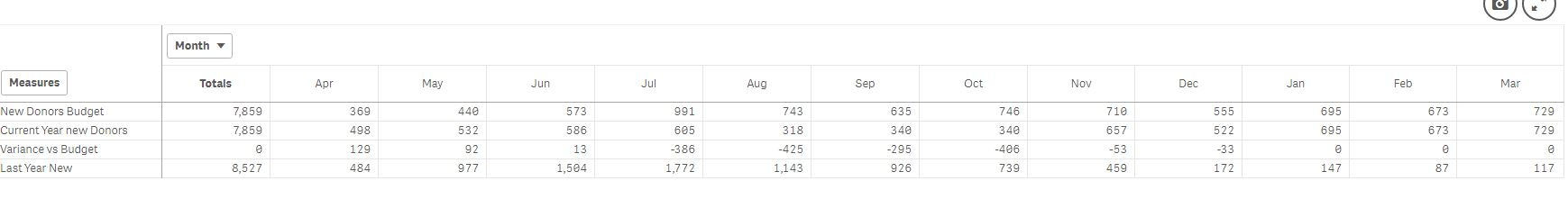# Qlik Sense App Development

Announcements
BI & Data Trends 2021. Discover the top 10 trends emerging in today. Join us on Dec. 8th REGISTER
cancel
Showing results for
Did you mean:
HighlightedCreator

## Pivot table totals

Hi all,

I created a set analysis for pivot table to calculate number of donors each moth. The formula displays actual number of donors in completed fiscal month but if a month not completed it displays forecast.

Here is a formula:

If(FiscalMonth < Today_FiscalMonth,

count({<FiscalYear = {'\$(=maxstring(FiscalYear))'},[Gift Type] = {[Recurring Gift Pay-Cash]}, DonorCurentSegment = {'New'}>} distinct [Constituent ID]),

sum({<DonortypeBDFC = {'New'}>} Numofdonors_Forecast))

It works fine for each month but as total id displays forecast summary instead of adding year to day actuals and forecast.

Can somebody help me to understand why it is happening?

Thanks

Denis

1 Solution

Accepted Solutions
HighlightedMVP

May be you need this:

Sum(Aggr(

If(FiscalMonth < Today_FiscalMonth,

count({<FiscalYear = {'\$(=maxstring(FiscalYear))'},[Gift Type] = {[Recurring Gift Pay-Cash]}, DonorCurentSegment = {'New'}>} distinct [Constituent ID]),

sum({<DonortypeBDFC = {'New'}>} Numofdonors_Forecast))

, Measures, Month))

6 Replies
HighlightedChampion II

can you post a snapshot of the pivot for more clarity

HighlightedCreator

Hi Vineeth,

Please see the snapshot belowHighlightedMVP

May be you need this:

Sum(Aggr(

If(FiscalMonth < Today_FiscalMonth,

count({<FiscalYear = {'\$(=maxstring(FiscalYear))'},[Gift Type] = {[Recurring Gift Pay-Cash]}, DonorCurentSegment = {'New'}>} distinct [Constituent ID]),

sum({<DonortypeBDFC = {'New'}>} Numofdonors_Forecast))

, Measures, Month))

HighlightedChampion II

try

SUM(

AGGR(

If(FiscalMonth < Today_FiscalMonth,

count({<FiscalYear = {'\$(=maxstring(FiscalYear))'},[Gift Type] = {[Recurring Gift Pay-Cash]}, DonorCurentSegment = {'New'}>} distinct [Constituent ID]),

sum({<DonortypeBDFC = {'New'}>} Numofdonors_Forecast))

,Measures,Month))

HighlightedCreator

Thanks a lot Sunny,

I totally forgot about aggregation function.

It worked thank you

HighlightedCreator

Thanks Vineeth!

It worked!